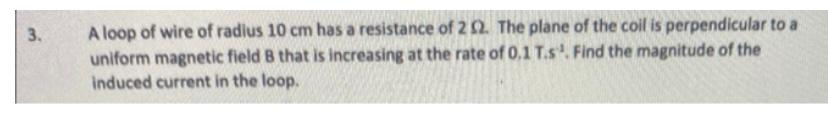# Question 3. A loop of wire of radius $$10 \mathrm{~cm}$$ has a resistance of $$2 \Omega$$. The plane of the coil is perpendicular to a uniform magnetic field $$B$$ that is increasing at the rate of $$0.1$$ T. $$\mathrm{s}^{-1}$$. Find the magnitude of the induced current in the loop. 3. A loop of wire of radius 10 cm has a resistance of 2Ω. The plane of the coil is perpendicular to a uniform magnetic field B that is increasing at the rate of 0.1 T. s−1. Find the magnitude of the induced current in the loop.